# 4. Logic Gates and Circuits

The functionality of even the most complex computer is achieved by connecting simple logic gates into operational circuits.

## Basic Gates

There are four basic logic gates. The symbols used for these gates and their "truth tables" are given below (note that these are not the "standard" electrical engineering symbols for these gates).

• NOT : unary input; output is reverse of input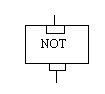INPUT OUTPUT 0 1 1 0

• AND : binary inputs: output is "on" only if both inputs are "on"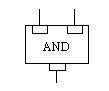INPUTS OUTPUT Input-1 Input-2 0 0 0 0 1 0 1 0 0 1 1 1

• OR : binary inputs: output is "off" only if both inputs are "off"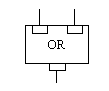INPUTS OUTPUT Input-1 Input-2 0 0 0 0 1 1 1 0 1 1 1 1

• XOR : binary inputs: output is "on" only if the inputs are different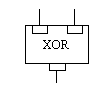INPUTS OUTPUT Input-1 Input-2 0 0 0 0 1 1 1 0 1 1 1 0

## Basic Binary Encoding

• requires a collection of circuits making up a "word"

• circuits are typically pictured a arranged in a horizontal row

• each circuit has a unique "weight" within the word

• right-most circuit has a weight of one (1)

• each remaining circuit has a weight which is twice as much as the weight of the circuit to its right.

• the number represented by a "word" is the sum of the weights of the circuits which are turned on.

Example (by common convention "0" is used to represent a circuit which is "off" and "1" is used to represent a circuit which is "on"):

• a "word" composed of 5 circuits (a 5 "bit" word) would have circuit weights of 16, 8, 4, 2, and 1 as viewed from left to right respectively

• if this word had a circuit pattern of 01001, then only the two circuits with weights 8 and 1 would be "on" and the number represented by the pattern in this word would be 9 (8+1)

0+0 = 0

0+1 = 1

1+0 = 1

1+1 = 10 (i.e. the result in this "column" is 0 with a "carry" of 1 that needs to be added to the next position to the left)

## Logic Gate Application for Binary Addition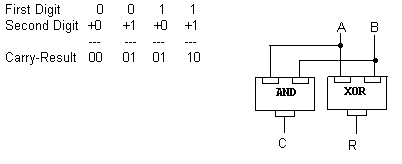Truth Table for Single Bit Addition:
 INPUTS OUTPUTS A B C R 0 0 0 0 0 1 0 1 1 0 0 1 1 1 1 0

Addition of "words" with multiple "bits" extends this logic.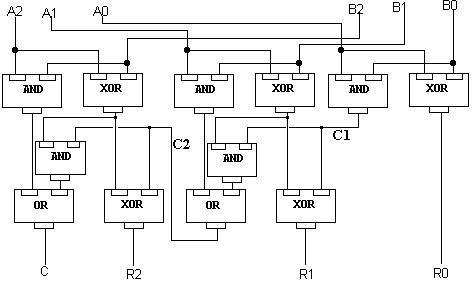## Selector

A collection of input wires or "bits" is "decoded" to turn on ("select") exactly one (output) circuit. (This is contrary to Englander's definition in "The Architecture of Computer Hardware and Software", page 720).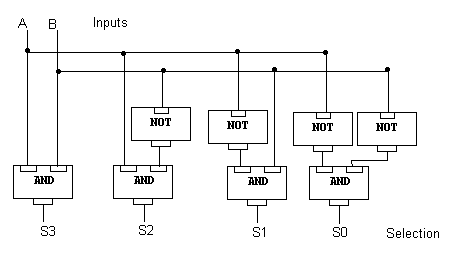## Multiplexor

A single input wire (at any one time) from a collection of possible inputs, is "encoded" into a pattern on a smaller collection of wires. A multiplexor performs the reverse function of a "selector". (The 2-input to 1-output ciruit described by Englander, as noted above, is a multiplexor).

## Memory Circuits

A single memory "bit" must have the ability
• to have a data value of either 0 or 1 "written" to it,
• to retain that value after the "write" request is done, and
• to return that retained value at a later time in response to a "read" request.
As another way of thinking about it:
• Sometimes the memory bit is Enabled (to be read from / written to); other times it is in a "passive" remembering state
• When enabled, a signal must indicate if we are trying to Read from it (the alternative being, write to it)
• If we are writing to it, an Input data value (0/1) must be provided
• If we are reading from it, an Output path must be provided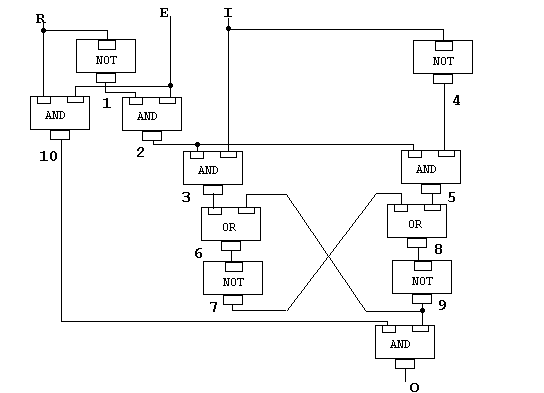Of course, to be very useful, we need many (millions) of these memory bits. A "Selector" circuit is then required to permit us to access a particular bit (or collection of bits) based on an input "address".